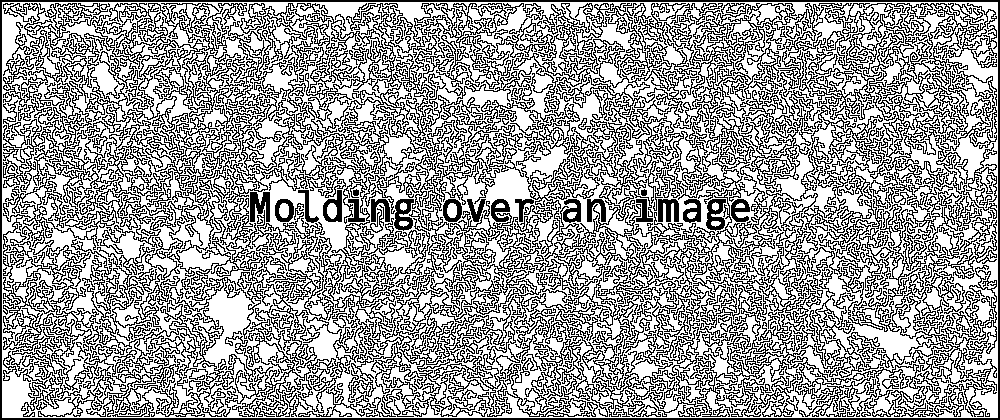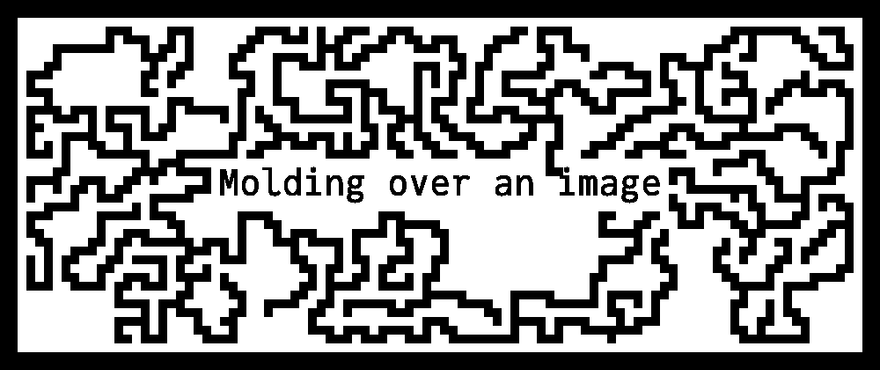## Julia Community 🟣David Gustavsson

Posted on • Updated on

# Custom array type for fake downsampling in Julia

I wrote a silly little algorithm that would "mold over" a black and white image -- I spawned a number of dots on the image, and if there was space to expand into a pixel without touching any already occupied pixles it would "consider" spreading to there. Every end spot with enough space would have a certain probability to move into a new spot, as well as a (lower) probability to branch into a second and third spot. Starting with the text "Molding over an image" on a blank canvas, for instance, lead to the cover image. This algorithm in itself wasn't very interesting, but there was one part of the problem that I felt showcased how easy certain things are with Julia:

My test images were all fairly low resolution, so I didn't notice when I first wrote the program, but it would make these really thin strands of mold and get really close to any other elements in the image, which in the end made those images difficult to read. I realized what I wanted to do was to downsample the image while running the algorithm (effectively making the mold thicker) and then upsample it again before saving, to recover the resolution of the original image elements.

In some other language, this might include editing the functions I'd written so far, but in Julia:

``````# "Hide" a boolean array inside a downsampled wrapper
struct DownsampledArray{T} <: AbstractArray{Bool,2}
data::T
k::Int64 # Downsampling level
function DownsampledArray(data::T, k) where {T}
if any(x -> ~iszero(rem(x, k)), size(data))
error("Can't downsample, size not divisible")
end
return new{T}(data, k)
end
end

function getindex(x::DownsampledArray, i::Vararg{Int,2})
# if any of the pixels in the block is true, consider the block true
return any(getindex(x.data, transformindex.(x.k, i)...))
end

function setindex!(x::DownsampledArray, v, i::Vararg{Int,2})
# Setting one pixel in the downsampled array sets all k by k pixels in the underlying array
return setindex!(x.data, fill(v, x.k, x.k), transformindex.(x.k, i)...)
end

transformindex(k, ind) = (k * (ind - 1) + 1):(k * ind)
size(x::DownsampledArray) = div.(size(x.data), x.k)
``````

This was quite quick to write, and this type could be hotswapped in for a normal `Matrix{Bool}`.

After running the function on this downsampled image, it could be upsampled again by simply extracting the underlying truth matrix - where the original elements were unaffected. The result for `k = 10` is shown below. Note the unchanged resolution on the text.This is of course a very specialized and mostly useless type - but that's why it's so cool: Would I have bothered to do a whole custom array type for a toy problem like this in another language? Would I have been able to do so while couch bound in a fever?

Anyway, it was inspiring to me. 🥔